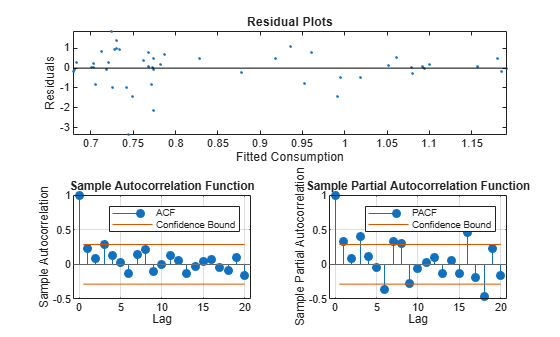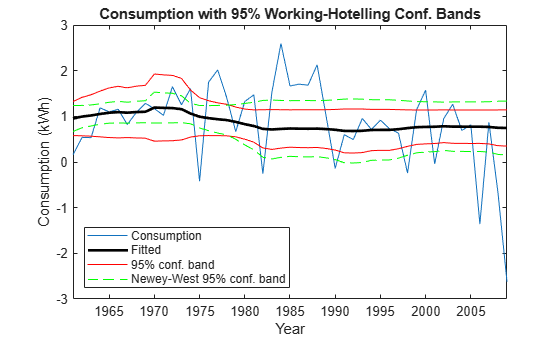Main Content

# Plot a Confidence Band Using HAC Estimates

This example shows how to plot heteroscedastic-and-autocorrelation consistent (HAC) corrected confidence bands using Newey-West robust standard errors.

One way to estimate the coefficients of a linear model is by OLS. However, time series models tend to have innovations that are autocorrelated and heteroscedastic (i.e., the errors are nonspherical). If a time series model has nonspherical errors, then usual formulae for standard errors of OLS coefficients are biased and inconsistent. Inference based on these inefficient standard errors tends to inflate the Type I error rate. One way to account for nonspherical errors is to use HAC standard errors. In particular, the Newey-West estimator of the OLS coefficient covariance is relatively robust against nonspherical errors.

### Load the Data

Load the Canadian electric power consumption data set from the World Bank. The response is Canada's electrical energy consumption in kWh (`DataTable.consump`), the predictor is Canada's GDP in year 2000 USD (`DataTable.gdp`), and the data set also contains the GDP deflator (`DataTable.gdp_deflator`). Because `DataTable` is a timetable, `DataTable.Time` is the sample year.

`load Data_PowerConsumption`

### Define the Model

Model the behavior of the annual difference in electrical energy consumption with respect to real GDP as a linear model:

`${\text{comsumpDiff}}_{t}={\beta }_{0}+{\beta }_{1}{\text{rGDP}}_{t}+{\epsilon }_{t}.$`

```consumpDiff = DataTable.consump - lagmatrix(DataTable.consump,1); ... % Annual difference in consumption T = size(consumpDiff,1); consumpDiff = consumpDiff(2:end)/1.0e+10; % Scale for numerical stability rGDP = DataTable.gdp./(DataTable.gdp_deflator); % Deflate GDP rGDP = rGDP(2:end)/1.0e+10; % Scale for numerical stability rGDPdes = [ones(T-1,1) rGDP]; % Design matrix Mdl = fitlm(rGDP,consumpDiff); coeff = Mdl.Coefficients(:,1); EstParamCov = Mdl.CoefficientCovariance; resid = Mdl.Residuals.Raw;```

### Plot the Data

Plot the difference in energy consumption, `consumpDiff` versus the real GDP, to check for possible heteroscedasticity.

```figure plot(rGDP,consumpDiff,'.') title 'Annual Difference in Energy Consumption vs real GDP - Canada'; xlabel 'real GDP (year 2000 USD)'; ylabel 'Annual Difference in Energy Consumption (kWh)';```The figure indicates that heteroscedasticity might be present in the annual difference in energy consumption. As real GDP increases, the annual difference in energy consumption seems to be less variable.

### Plot the residuals.

Plot the residuals from `Mdl` against the fitted values and year to assess heteroscedasticity and autocorrelation.

```figure subplot(2,1,1) hold on plot(Mdl.Fitted,resid,'.') plot([min(Mdl.Fitted) max(Mdl.Fitted)],[0 0],'k-') title 'Residual Plots'; xlabel 'Fitted Consumption'; ylabel 'Residuals'; axis tight hold off subplot(2,2,3) autocorr(resid) h1 = gca; h1.FontSize = 8; subplot(2,2,4) parcorr(resid) h2 = gca; h2.FontSize = 8;```The residual plot reveals decreasing residual variance with increasing fitted consumption. The autocorrelation function shows that autocorrelation might be present in the first few lagged residuals.

### Test for heteroscedasticity and autocorrelation.

Test for conditional heteroscedasticity using Engle's ARCH test. Test for autocorrelation using the Ljung-Box Q test. Test for overall correlation using the Durbin-Watson test.

```[~,englePValue] = archtest(resid); englePValue```
```englePValue = 0.1463 ```
```[~,lbqPValue] = lbqtest(resid,'lags',1:3);... % Significance of first three lags lbqPValue```
```lbqPValue = 1×3 0.0905 0.1966 0.0522 ```
```[dwPValue] = dwtest(Mdl); dwPValue```
```dwPValue = 0.0024 ```

The p value of Engle's ARCH test suggests significant conditional heteroscedasticity at 15% significance level. The p value for the Ljung-Box Q test suggests significant autocorrelation with the first and third lagged residuals at 10% significance level. The p value for the Durbin-Watson test suggests that there is strong evidence for overall residual autocorrelation. The results of the tests suggest that the standard linear model conditions of homoscedasticity and uncorrelated errors are violated, and inferences based on the OLS coefficient covariance matrix are suspect.

One way to proceed with inference (such as constructing a confidence band) is to correct the OLS coefficient covariance matrix by estimating the Newey-West coefficient covariance.

### Estimate the Newey-West coefficient covariance.

Correct the OLS coefficient covariance matrix by estimating the Newey-West coefficient covariance using `hac`. Compute the maximum lag to be weighted for the standard Newey-West estimate, `maxLag` (Newey and West, 1994). Use `hac` to estimate the standard Newey-West coefficient covariance.

```maxLag = floor(4*(T/100)^(2/9)); [NWEstParamCov,~,NWCoeff] = hac(Mdl,'type','hac',... 'bandwidth',maxLag + 1);```
```Estimator type: HAC Estimation method: BT Bandwidth: 4.0000 Whitening order: 0 Effective sample size: 49 Small sample correction: on Coefficient Covariances: | Const x1 -------------------------- Const | 0.3720 -0.2990 x1 | -0.2990 0.2454 ```

The Newey-West standard error for the coefficient of `rGDP`, labeled ${x}_{1}$ in the table, is less than the usual OLS standard error. This suggests that, in this data set, correcting for residual heteroscedasticity and autocorrelation increases the precision in measuring the linear effect of real GDP on energy consumption.

### Calculate the Working-Hotelling confidence bands.

Compute the 95% Working-Hotelling confidence band for each covariance estimate using `nlpredci` (Kutner et al., 2005).

```modelfun = @(b,x)(b(1)*x(:,1)+b(2)*x(:,2)); % Define the linear model [beta,nlresid,~,EstParamCov] = nlinfit(rGDPdes,... consumpDiff,modelfun,[1,1]); % estimate the model [fity,fitcb] = nlpredci(modelfun,rGDPdes,beta,nlresid,... 'Covar',EstParamCov,'SimOpt','on'); % Margin of errors conbandnl = [fity - fitcb fity + fitcb]; % Confidence bands [fity,NWfitcb] = nlpredci(modelfun,rGDPdes,... beta,nlresid,'Covar',NWEstParamCov,'SimOpt','on'); % Corrected margin of error NWconbandnl = [fity - NWfitcb fity + NWfitcb]; % Corrected confidence bands```

### Plot the Working-Hotelling confidence bands.

Plot the Working-Hotelling confidence bands on the same axes twice: one plot displaying electrical energy consumption with respect to real GDP, and the other displaying the electrical energy consumption time series.

```figure hold on l1 = plot(rGDP,consumpDiff,'k.'); l2 = plot(rGDP,fity,'b-','LineWidth',2); l3 = plot(rGDP,conbandnl,'r-'); l4 = plot(rGDP,NWconbandnl,'g--'); title 'Data with 95% Working-Hotelling Conf. Bands'; xlabel 'real GDP (year 2000 USD)'; ylabel 'Consumption (kWh)'; axis([0.7 1.4 -2 2.5]) legend([l1 l2 l3(1) l4(1)],'Data','Fitted','95% Conf. Band',... 'Newey-West 95% Conf. Band','Location','SouthEast') hold off``````figure year = DataTable.Time(2:end); hold on l1 = plot(year,consumpDiff); l2 = plot(year,fity,'k-','LineWidth',2); l3 = plot(year,conbandnl,'r-'); l4 = plot(year,NWconbandnl,'g--'); title 'Consumption with 95% Working-Hotelling Conf. Bands'; xlabel 'Year'; ylabel 'Consumption (kWh)'; legend([l1 l2 l3(1) l4(1)],'Consumption','Fitted',... '95% Conf. Band','Newey-West 95% Conf. Band',... 'Location','SouthWest') hold off```The plots show that the Newey-West estimator accounts for the heteroscedasticity in that the confidence band is wide in areas of high volatility, and thin in areas of low volatility. The OLS coefficient covariance estimator ignores this pattern of volatility.

References:

1. Kutner, M. H., C. J. Nachtsheim, J. Neter, and W. Li. Applied Linear Statistical Models. 5th Ed. New York: McGraw-Hill/Irwin, 2005.

2. Newey, W. K., and K. D. West. "A Simple Positive Semidefinite, Heteroskedasticity and Autocorrelation Consistent Covariance Matrix." Econometrica. Vol. 55, 1987, pp. 703-708.

3. Newey, W. K, and K. D. West. "Automatic Lag Selection in Covariance Matrix Estimation." The Review of Economic Studies. Vol. 61 No. 4, 1994, pp. 631-653.

Download ebook